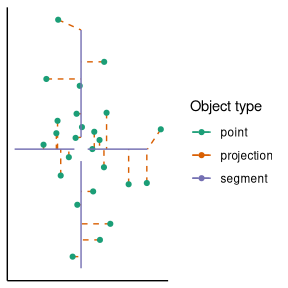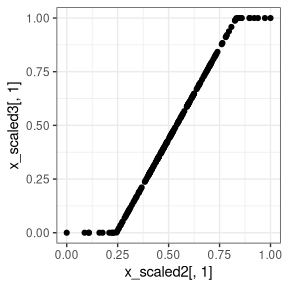# Overview of the functionality provided by the dynutils package

## Manipulation of lists

### add_class: Add a class to an object

l <- list(important_number = 42) %>% add_class("my_list")
l
#>  42
#>
#> $url #>  "https://github.com/dynverse/dynverse" #> #> attr(,"class") #>  "improved_list" "my_list" "list" ## Calculations ### calculate_distance: Compute pairwise distances between two matrices See ?calculate_distance for the list of currently supported distances. x <- matrix(runif(30), ncol = 10) y <- matrix(runif(50), ncol = 10) calculate_distance(x, y, method = "euclidean") #> [,1] [,2] [,3] [,4] [,5] #> [1,] 1.184305 0.9571034 1.1284052 1.022205 1.5531565 #> [2,] 1.078353 1.1378581 0.7076092 1.072164 0.8846294 #> [3,] 1.335627 1.4116406 1.3526085 1.239853 1.6282805 For euclidean distances, this is similar to calculating: as.matrix(dist(rbind(x, y)))[1:3, -1:-3] #> 4 5 6 7 8 #> 1 1.184305 0.9571034 1.1284052 1.022205 1.5531565 #> 2 1.078353 1.1378581 0.7076092 1.072164 0.8846294 #> 3 1.335627 1.4116406 1.3526085 1.239853 1.6282805 ### project_to_segments: Project a set of points to to set of segments x <- matrix(rnorm(50, 0, .5), ncol = 2) segfrom <- matrix(c(0, 1, 0, -1, 1, 0, -1, 0), ncol = 2, byrow = TRUE) segto <- segfrom / 10 fit <- project_to_segments(x, segfrom, segto) ggplot() + geom_segment(aes(x = x[,1], xend = fit$x_proj[,1], y = x[,2], yend = fit$x_proj[,2], colour = "projection"), linetype = "dashed") + geom_point(aes(x[,1], x[,2], colour = "point")) + geom_segment(aes(x = segfrom[,1], xend = segto[,1], y = segfrom[,2], yend = segto[,2], colour = "segment")) + scale_colour_brewer(palette = "Dark2") + scale_x_continuous(name = NULL, breaks = NULL) + scale_y_continuous(name = NULL, breaks = NULL) + labs(colour = "Object type") + theme_classic()str(fit) #> List of 4 #>$ x_proj     : num [1:25, 1:2] 0 0 0 0.278 0 ...
#>  $distance : num [1:25] 0.0068 0.01605 0.12144 0.00587 0.12604 ... #>$ segment    : int [1:25] 1 2 1 3 1 4 2 3 2 2 ...
#>  $progression: num [1:25] 1 0.108 0.297 0.802 0 ... ### calculate_mean: Calculate a (weighted) mean between vectors or a list of vectors; supports the arithmetic, geometric and harmonic mean calculate_arithmetic_mean(0.1, 0.5, 0.9) #>  0.5 calculate_geometric_mean(0.1, 0.5, 0.9) #>  0.3556893 calculate_harmonic_mean(0.1, 0.5, 0.9) #>  0.2288136 calculate_mean(.1, .5, .9, method = "harmonic") #>  0.2288136 # example with multiple vectors calculate_arithmetic_mean(c(0.1, 0.9), c(0.2, 1)) #>  0.15 0.95 # example with a list of vectors vectors <- list(c(0.1, 0.2), c(0.4, 0.5)) calculate_geometric_mean(vectors) #>  0.2000000 0.3162278 # example of weighted means calculate_geometric_mean(c(0.1, 10), c(0.9, 20), c(0.5, 2), weights = c(1, 2, 5)) #>  0.4736057 4.3491186 ## Manipulation of matrices ### expand_matrix: Add rows and columns to a matrix x <- matrix(runif(12), ncol = 4, dimnames = list(c("a", "c", "d"), c("D", "F", "H", "I"))) expand_matrix(x, letters[1:5], LETTERS[1:10], fill = 0) #> A B C D E F G H I J #> a 0 0 0 0.2937302 0 0.5033395 0 0.7581031 0.5476466 0 #> b 0 0 0 0.0000000 0 0.0000000 0 0.0000000 0.0000000 0 #> c 0 0 0 0.1912601 0 0.8770575 0 0.7244989 0.7117439 0 #> d 0 0 0 0.8864509 0 0.1891936 0 0.9437248 0.3889051 0 #> e 0 0 0 0.0000000 0 0.0000000 0 0.0000000 0.0000000 0 ## Scaling of matrices and vectors ### scale_uniform: Rescale data to have a certain center and max range Generate a matrix from a normal distribution with a large standard deviation, centered at c(5, 5). x <- matrix(rnorm(200*2, sd = 10, mean = 5), ncol = 2) Center the dataset at c(0, 0) with a minimum of c(-.5, -.5) and a maximum of c(.5, .5). x_scaled <- scale_uniform(x, center = 0, max_range = 1) Check the ranges and verify that the scaling is correct. ranges <- apply(x_scaled, 2, range) ranges # should all lie between -.5 and .5 #> [,1] [,2] #> [1,] -0.4061179 -0.5 #> [2,] 0.4061179 0.5 colMeans(ranges) # should all be equal to 0 #>  0 0 apply(ranges, 2, diff) # max should be 1 #>  0.8122358 1.0000000 ### scale_minmax: Rescale data to a [0, 1] range x_scaled2 <- scale_minmax(x) Check the ranges and verify that the scaling is correct. apply(x_scaled2, 2, range) # each column should be [0, 1] #> [,1] [,2] #> [1,] 0 0 #> [2,] 1 1 ### scale_quantile: Cut off outer quantiles and rescale to a [0, 1] range x_scaled3 <- scale_quantile(x, .05) Check the ranges and verify that the scaling is correct. apply(x_scaled3, 2, range) # each column should be [0, 1] #> [,1] [,2] #> [1,] 0 0 #> [2,] 1 1 qplot(x_scaled2[,1], x_scaled3[,1]) + theme_bw()## Manipulation of functions ### inherit_default_params: Have one function inherit the default parameters from other functions fun1 <- function(a = 10, b = 7) runif(a, -b, b) fun2 <- function(c = 9) 2^c fun3 <- inherit_default_params( super = list(fun1, fun2), fun = function(a, b, c) { list(x = fun1(a, b), y = fun2(c)) } ) fun3 #> function (a = 10, b = 7, c = 9) #> { #> list(x = fun1(a, b), y = fun2(c)) #> } ## Manipulation of packages ### check_packages: Easily checking whether certain packages are installed check_packages("SCORPIUS", "dynutils", "wubbalubbadubdub") #> SCORPIUS dynutils wubbalubbadubdub #> FALSE TRUE FALSE check_packages(c("princurve", "mlr", "tidyverse")) #> princurve mlr tidyverse #> FALSE FALSE TRUE ### install_packages: Install packages taking into account the remotes of another This is useful for installing suggested packages with GitHub remotes. install_packages("SCORPIUS", package = "dynmethods", prompt = TRUE) > install_packages("SCORPIUS", package = "dynmethods", prompt = TRUE) Following packages have to be installed: SCORPIUS Do you want to install these packages? (y/yes/1 or n/no/2): 1 Installing SCORPIUS ... ** testing if installed package can be loaded * DONE (SCORPIUS) Installed SCORPIUS  "SCORPIUS" ## Manipulation of vectors ### random_time_string: Generates a string very likely to be unique random_time_string("test") #>  "20220923_061947__test__syYeN626jT" random_time_string("test") #>  "20220923_061947__test__e6MCnXcJM7" random_time_string("test") #>  "20220923_061947__test__9pFJ5mxS3R" ## Tibble helpers ### list_as_tibble: Convert a list of lists to a tibble whilst retaining class information li <- list( list(a = 1, b = log10, c = "parrot") %>% add_class("myobject"), list(a = 2, b = sqrt, c = "quest") %>% add_class("yourobject") ) tib <- list_as_tibble(li) tib #> # A tibble: 2 × 4 #> a b c .object_class #> <dbl> <list> <chr> <list> #> 1 1 <fn> parrot <chr > #> 2 2 <fn> quest <chr > ### tibble_as_list: Convert a tibble back to a list of lists whilst retaining class information li <- tibble_as_list(tib) li #> [] #>$a
#>  1
#>
#> $b #> function (x) .Primitive("log10") #> #>$c
#>  "parrot"
#>
#> attr(,"class")
#>  "myobject" "list"
#>
#> []
#> $a #>  2 #> #>$b
#> function (x)  .Primitive("sqrt")
#>
#> $c #>  "quest" #> #> attr(,"class") #>  "yourobject" "list" ### extract_row_to_list: Extracts one row from a tibble and converts it to a list extract_row_to_list(tib, 2) #>$a
#>  2
#>
#> $b #> function (x) .Primitive("sqrt") #> #>$c
#>  "quest"
#>
#> attr(,"class")
#>  "yourobject" "list"

### mapdf: Apply a function to each row of a data frame

The mapdf functions apply a function on each row of a data frame. They are based heavily on purrr’s map functions.

tib %>% mapdf(class)
#> []
#>  "myobject" "list"
#>
#> []
#>  "yourobject" "list"

Or use an anonymous function.

tib %>% mapdf(function(row) paste0(row$b(row$a), "_", row$c)) #> [] #>  "0_parrot" #> #> [] #>  "1.4142135623731_quest" Or even a formula. tib %>% mapdf(~ .$b)
#> []
#> function (x)  .Primitive("log10")
#>
#> []
#> function (x)  .Primitive("sqrt")

There are many more variations available. See ?mapdf for more info.

tib %>% mapdf_lgl(~ .$a > 1) #>  FALSE TRUE tib %>% mapdf_chr(~ paste0("~", .$c, "~"))
#>  "~parrot~" "~quest~"
tib %>% mapdf_int(~ nchar(.$c)) #>  6 5 tib %>% mapdf_dbl(~ .$a * 1.234)
#>  1.234 2.468

## File helpers

### safe_tempdir: Create an empty temporary directory and return its path

safe_tempdir("samson")
#>  "/tmp/RtmpsKiNj7/file28419731ffb2cf/samson"

## Assertion helpers

### %all_in%: Check whether a vector are all elements of another vector

library(assertthat)
assert_that(c(1, 2) %all_in% c(0, 1, 2, 3, 4))
#>  TRUE
assert_that("a" %all_in% letters)
#>  TRUE
assert_that("A" %all_in% letters)
#> Error: "A" is missing 1 element from letters: "A"
assert_that(1:10 %all_in% letters)
#> Error: 1:10 is missing 10 elements from letters: 1L, 2L, 3L, ...

### %has_names%: Check whether an object has certain names

assert_that(li %has_names% "a")
#> Error: li is missing 1 name from "a": "a"
assert_that(li %has_names% "c")
#> Error: li is missing 1 name from "c": "c"
assert_that(li %has_names% letters)
#> Error: li is missing 26 names from letters: "a", "b", "c", ...

### is_single_numeric: Check whether a value is a single numeric

assert_that(is_single_numeric(1))
#>  TRUE
assert_that(is_single_numeric(Inf))
#>  TRUE
assert_that(is_single_numeric(1.6))
#>  TRUE
assert_that(is_single_numeric(NA))
#> Error: NA is not a single numeric value
assert_that(is_single_numeric(1:6))
#> Error: 1:6 is not a single numeric value
assert_that(is_single_numeric("pie"))
#> Error: "pie" is not a single numeric value

### is_bounded: Check whether a value within a certain interval

assert_that(is_bounded(10))
#>  TRUE
assert_that(is_bounded(10:30))
#>  TRUE
assert_that(is_bounded(Inf))
#> Error: Inf is not bounded by (-Inf,Inf)
assert_that(is_bounded(10, lower_bound = 20))
#> Error: 10 is not bounded by (20,Inf)
assert_that(is_bounded(
10,
lower_bound = 20,
lower_closed = TRUE,
upper_bound = 30,
upper_closed = FALSE
))
#> Error: 10 is not bounded by [20,30)